Fatigue Index

Introduction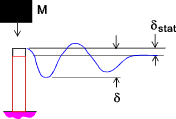It is normal practice to design machines such that impact loads are eliminated or reduced by inclusion of shock absorbers.  Inclusion of low cost, mass produced, shock absorbers can virtually eliminate the increased stresses and deformations resulting from impact loads.

Most ductile materials have strength properties which are a function of the loading speed.   The more rapid the loading the higher the tensile and ultimate strengths of the materials.   .  Two standard tests, the Charpy and Izod, measure the impact energy (the energy required to fracture a test piece under an impact load), also called the notch toughness.

The detailed assessment of the strength of machine elements under impact loading regimes involves use of advanced techniques including Finite Element Analysis.   Impact loads result in shock waves propogating through the elements with possible serious consequences.  It is possible to complete a relatively simply stress evaluation for suddenly applied and impact loads by using the principle of conservation of energy and conditional that the materials considered are operating within their elastic regions.

The equations are most accurate for relatively heavy impacting masses moving at low velocities on impact.

Notation

 A = Area (m2) E = Modulus of Elasticity (N/m2) h = Drop distance (m) k =Stiffness (N/m) M = Mass-moving body (kg) M1 = Mass of static bar or beam (kg) K = Factor to allow for energy loss at impact l = length of bar (l) tp = time period (s) v = velocity (m/s) W = Weight - moving body(N) V = Velocity (m/s) w = Specific Weight (kg/m3) σ = stress (N/m2) σ stat = stress resulting from static load(N/m2) δ = deflection (m) δ stat = deflection resulting from static load(m) μ = Ratio Moving Mass/Stationary bar β = Constant = A Sqrt(wEg/W) - see text

Linear Impact deflections and stresses (gravity loads)

Important note: The notes below represent a very simple view of the loading condition and do not consider more real case involving shock waves being propagated through the loaded member or the moving mass

Consider a loading regime as shown below with a ring of Mass M(kg) with weight W= M.g(N) being dropped through a distance h onto a collar supported by a vertical bar which behaves as a spring with a stiffness of k (N/m).

The support bar has a length l (m), an Area A (m2 ) With a modulus of elasticity E (N/m2 )

In practice the weight would impact onto the support which would elastically deform until all of the potential energy has been absorbed.  The support would then contract initiating damped oscillations until the system assumes a stable static position.  The equations below determine the initial maximum deformation which provides the most highly stressed condition.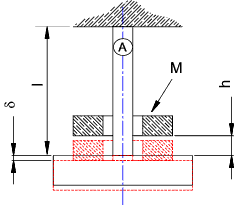In accordance with conservation of energy the potential energy of the weight is converted to elastic strain energy.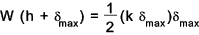This may be expressed as a quadratic equation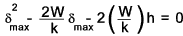This is solved for the maximum deflection δmax as follows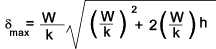The weight applied gradually would result in a deflection δst thus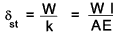Note:
The stiffness k = Force /Deflection = F /δ:    E = stress /strain = σ /e = (F /A) /(δ/l)    Therefore k = EA/l

Substituting this into the equation for δmax results in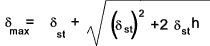This can be expressed as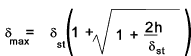The resultant maximum force is simply

Pmax = k δmax

and the resultant maximum stress =

σmax = Pmax/ A

This may be expressed as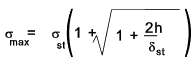For the calculation of the stress due to a suddenly applied load with h = 0

σmax = 2 σst

Linear impact deflections and stresses (kinetic impacts )

Important note: The notes below represent a very simple view of the loading condition and do not consider more real case involving shock waves being propagated through the loaded member or the moving mass

Impact loads based pimarily on kinetic energy e.g horizontal impacts are treated slightly differently.  For these applications the kinetic energy is converted into stored energy due to elasticity of the resisting element.

Consider a Mass M(kg) with a velocity of v impacting on a collar which is supported by a bar with a stiffness of K (N/m) - Ignoring gravitational forces.

The kinetic energy of the mass Mv2 /2 is transformed into stored energy in the support.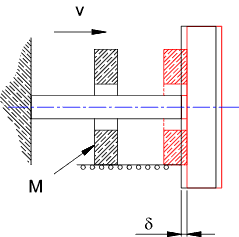The resulting equation is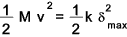The resultant maximum deflection equals..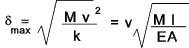Noting that the static deflection = Wl/AE this equation can be written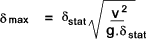The equivalent maximum stress =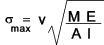Noting that the static deflection = Wl/AE this equation can be written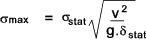Beams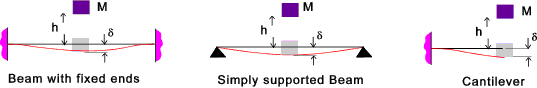Using similar principles as expressed above it can be easily proved using principles of conservation of energy that.andIf the impact is horizontal instead of vertical . The resulting deformation and stress resulting from the impact areNote: The above approximate relationships can been applied generally to most structural systems subject to distortion with the elastic range when subject to impact loading

Energy losses on impact

The above equations are very approximate and include many assumptions.  A very important assumption is that all of the energy ( based on h or v2) is used up in producing the same distortion as would result from static loading.  In reality, some kinetic energy is lost in internal friction.  Account can be taken for these losses by multiplying h or v2 by an appropriate factor K.  This factor is derived from the Mass of the moving body (M) and the mass of the beam or bar (M1).  The factor is different for different loading systems ass follows.

In the equations above h or v2 would be replaced by K.h or K v2
The K factor is nearest unity when M is large compared to M1 . As an example for the axial impact

if M1/M is say 0,1 then K = 0,95..
if M1/M is say 10 then K = 0,15..

This is illustrated in the figure below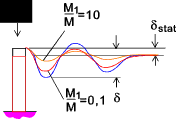1) A moving mass M striking axially one end of a bar of mass M1. The other end of the bar being fixed...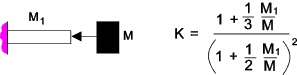2) A moving mass M striking traversely the end of a beam of mass M1...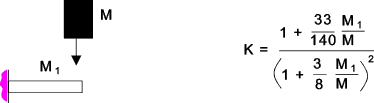3) A moving mass M striking traversely the center of a beam with simple supported ends of mass M1....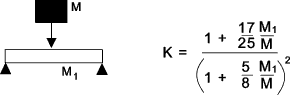4) A moving mass M striking traversely the center of a beam with fixed ends of mass M1. ..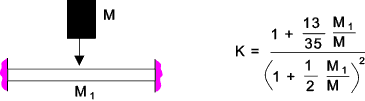Impact stresses considering propagation of shock waves- Unsupported bar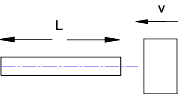When a impact force is suddenly applied to and elastic body , a wave of stress is propogated traveling through the body with a velocity..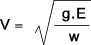w = weight /unit volume (kg/m2), v = velocity (m/s)

The unsupported bar subject to the longitudonal impact from a rigid body with velocity v experiences a wave of compressive stress of intensity σ.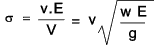If the mass of the moving body is very large compared to the mass of the bar the wave of compression bounces back from the far end of the bar as a wave of tension and returns to the struck end after a time period .

t p = 2L / V

If the mass of the moving body is very large compared to the bar so that it can be considered infinite then after breaking contact the moving bar will move away from the impacting mass with a velocity of vb= 2v.   The moving bar will be stress free.

If the mass of the impinging body is μ time the mass of the bar then the bar will move away with an average velocity of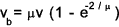The moving bar is left vibrating with a stress intensity of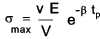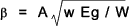Impacts considering propagation of shock waves- bar with one end supported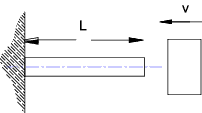For the case of a bar with one end fixed , the wave of compressive stress resulting from the impact on the unsupported end is reflected back unchanged from the supported (fixed end) and combines with the advancing waves to produce a maximum stress approximately equal to..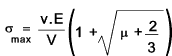μ = Mass of Moving Body/Mass of Bar